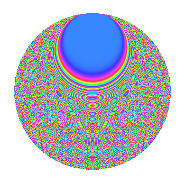# Properties

 Label 2365.2.bnLevel 2365 Weight 2 Character orbit bn Rep. character $$\chi_{2365}(914,\cdot)$$ Character field $$\Q(\zeta_{14})$$ Dimension 1320 Sturm bound 528

# Related objects

## Defining parameters

 Level: $$N$$ = $$2365 = 5 \cdot 11 \cdot 43$$ Weight: $$k$$ = $$2$$ Character orbit: $$[\chi]$$ = 2365.bn (of order $$14$$ and degree $$6$$) Character conductor: $$\operatorname{cond}(\chi)$$ = $$215$$ Character field: $$\Q(\zeta_{14})$$ Sturm bound: $$528$$

## Dimensions

The following table gives the dimensions of various subspaces of $$M_{2}(2365, [\chi])$$.

Total New Old
Modular forms 1608 1320 288
Cusp forms 1560 1320 240
Eisenstein series 48 0 48

## Trace form

 $$1320q + 220q^{4} - 8q^{6} + 200q^{9} + O(q^{10})$$ $$1320q + 220q^{4} - 8q^{6} + 200q^{9} - 12q^{14} - 200q^{16} + 16q^{19} + 28q^{20} - 48q^{21} - 32q^{24} - 20q^{25} + 16q^{26} + 72q^{29} + 104q^{30} + 32q^{31} - 8q^{34} - 28q^{35} + 1376q^{36} - 24q^{39} - 40q^{40} - 32q^{41} + 8q^{44} - 20q^{45} + 56q^{46} - 1272q^{49} - 120q^{50} + 128q^{51} - 72q^{54} + 24q^{56} + 16q^{59} + 140q^{60} - 48q^{61} + 236q^{64} - 12q^{65} - 12q^{69} + 112q^{70} + 8q^{71} - 64q^{74} - 140q^{75} - 96q^{76} - 72q^{79} - 112q^{80} - 120q^{81} - 36q^{84} + 60q^{85} + 104q^{86} - 36q^{89} - 14q^{90} - 64q^{91} - 120q^{94} + 80q^{95} - 328q^{96} + O(q^{100})$$

## Decomposition of $$S_{2}^{\mathrm{new}}(2365, [\chi])$$ into newform subspaces

The newforms in this space have not yet been added to the LMFDB.

## Decomposition of $$S_{2}^{\mathrm{old}}(2365, [\chi])$$ into lower level spaces

$$S_{2}^{\mathrm{old}}(2365, [\chi]) \cong$$ $$S_{2}^{\mathrm{new}}(215, [\chi])$$$$^{\oplus 2}$$

## Hecke Characteristic Polynomials

There are no characteristic polynomials of Hecke operators in the database